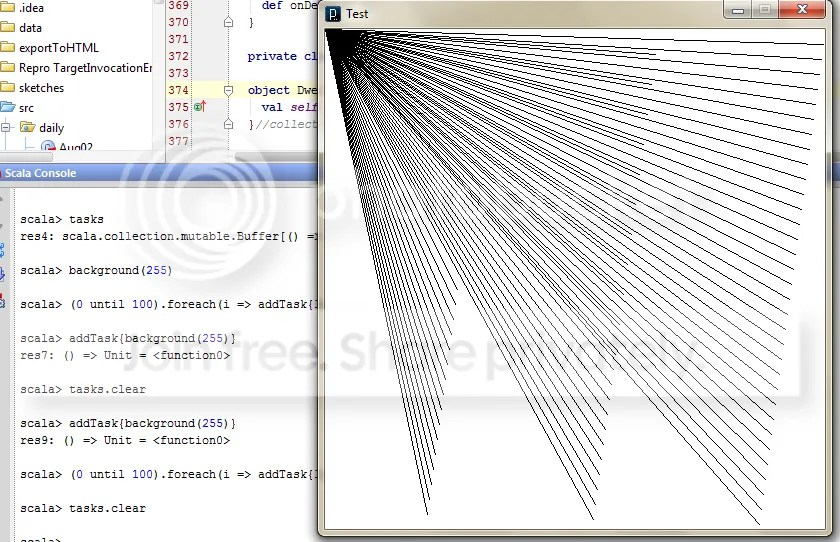# Processing in Scala REPL

Assuming you’ve got processing in your classpath, you can now interactively draw and compose sketches through the REPL using this piece of code.

### 2D version as of [08/09/2011] (for 2D):

```object Scheduler { val tasks = collection.mutable.Buffer[() => Any]();  def addTask[A](t: => A):() => A = { lazy val task = {() => t}; tasks += task; task }; def removeTask[A](t: () => A) {tasks -= t} }
import processing.core._; import PApplet._; import PConstants._; import Scheduler._;
object Test extends PApplet {
override def setup() { size(500, 500); }
}
PApplet.runSketch(Array("Test"), Test)
import Test._```

### 3D version as of [08/10/2011] (using PeasyCam):

```object Scheduler { val tasks = collection.mutable.Buffer[() => Any]();  def addTask[A](t: => A):() => A = { lazy val task = {() => t}; tasks += task; task }; def removeTask[A](t: () => A) {tasks -= t} }
import processing.core._; import PApplet._; import PConstants._; import Scheduler._;
object Test extends PApplet {
override def setup() { size(500, 500, P3D); new peasy.PeasyCam(this, 600); }
}
PApplet.runSketch(Array("Test"), Test)
import Test._```

#### Changelog:

[08/10/2011] – Typo in 3D version fixed.

[08/09/2011] – Added a Scheduler. You may add and remove arbitrary code blocks to be executed during draw(); e.g.

```scala> addTask{ ellipse(random(width), random(height), 25, 25); } //this effectively puts the ellipse call inside draw
res1: () => Unit = <function0>

scala> removeTask(res1) //stop drawing random ellipses
scala> tasks //tasks is just a Buffer that you can change at will
res4: scala.collection.mutable.Buffer[() => Any] = ArrayBuffer()

res7: () => Unit = <function0>1.Interactive Processing Window from REPL « Processing with Scala says: